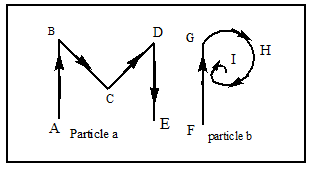Choose the correct statement by observing the following figure:1) The path of particle (a) is ABCD 2) The path of particle (b) is FGHI3) The path of particle (a) is ABCDE 4) The path of particle (b) is FGH

# Choose the correct statement by observing the following figure:1) The path of particle (a) is ABCD 2) The path of particle (b) is FGHI3) The path of particle (a) is ABCDE 4) The path of particle (b) is FGH

1. A

1, 2 and 3 are true

2. B

1 and 2 are true

3. C

1 and 3 are true

4. D

2 and 3 are true

Register to Get Free Mock Test and Study Material

+91

Verify OTP Code (required)

### Solution:

By conceptualRegister to Get Free Mock Test and Study Material

+91

Verify OTP Code (required)